« Vrati se

#### IMO Shortlist 1999 problem G8

Given a triangle$ABC$. The points$A$,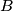$B$,$C$ divide the circumcircle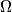$\Omega$ of the triangle$ABC$ into three arcs$BC$,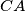$CA$,$AB$. Let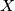$X$ be a variable point on the arc$AB$, and let$O_{1}$ and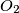$O_{2}$ be the incenters of the triangles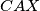$CAX$ and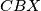$CBX$. Prove that the circumcircle of the triangle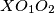$XO_{1}O_{2}$ intersects the circle$\Omega$ in a fixed point.